## Graph Spectral Compressed Sensing

### Graph Spectral Compressed Sensing

Project Overview:

In this project, we consider a signal whose entries are supported on the nodes of a graph. We study the metric to measure the smoothness of signals supported on graphs and provide theoretical explanations for when and why the Laplacian eigenbasis can be regarded as a meaningful "Fourier" transform of such signals. Moreover, we characterize the desired properties of the underlying graph for better compressibility of the signals. For a smooth signal with respect to the graph topology, our work proves that we can gather measurements from a random subset of nodes and then obtain a stable recovery with respect to the graph Laplacian eigenbasis, leveraging ideas from compressed sensing. We also show how such techniques can be used for both temporally and spatially correlated signals sampled by wireless sensor networks. Significant savings are made in terms of energy resources, bandwidth, and query latency by using this approach. All the theoretical analysis and the performance of proposed algorithms are verified using both synthesized data and real world data.

Signals supported on graphs:

Signals supported on graphs are fairly common in real applications. When we say a signal is supported on graph, we mean that each entry of the signal is supported on the vertices of a graph. In this project, we are very interested in the situation that the distribution of the signal is close related to its underlying graph topology. For example, the following figure shows one example of the signals supported on graphs: the nodes in the figure represent the weather sensor distributed in the state of California and its color corresponds to the solar radiation reading. We construct the underlying graph via a KNN graph based on the location of the nodes.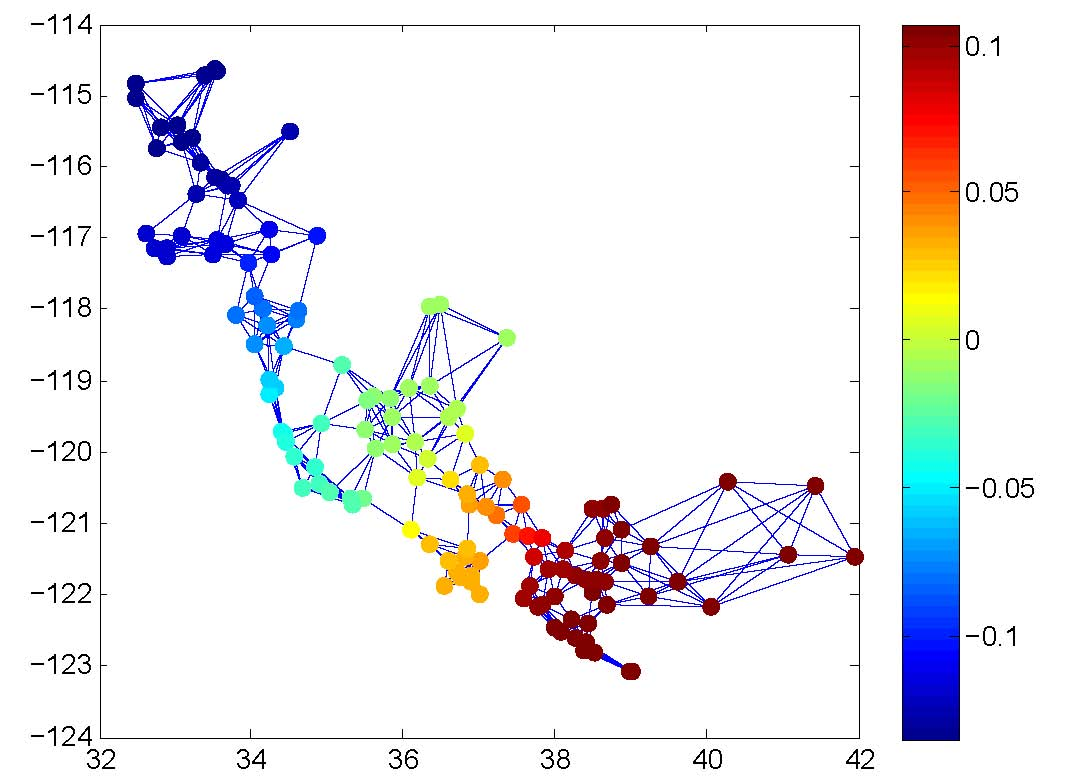For conventional signals like speech, audio or images, a highly developed theory called approximation theory can be utilized to deal with such signals of regular structure and technologies like JPEG or MP3 based on approximation theory helps reduce the overhead if we want to transmit the conventional signals. Unlike the conventional approximation theory, we are interested in the signals supported on graphs, i.e., signals of irregular structure. We try to answer the question: how can we approximate signals on graphs?

Graph Fourier Transform:

One main contribution of this project is that we make a theoretical analysis to show that the graph Laplacian eigenbasis can be regarded as the "Graph Fourier Transform (GFT)". We first study the concept of smooth signals and make a detailed analysis of the metric to measure the smoothness of a graph signal. Later, we derive certain properties of the GFT. Those properties imply that if the eigenvalues of the graph Laplacian roughly maintain an increasing trend, then the smooth signals on that graph are likely to be compressible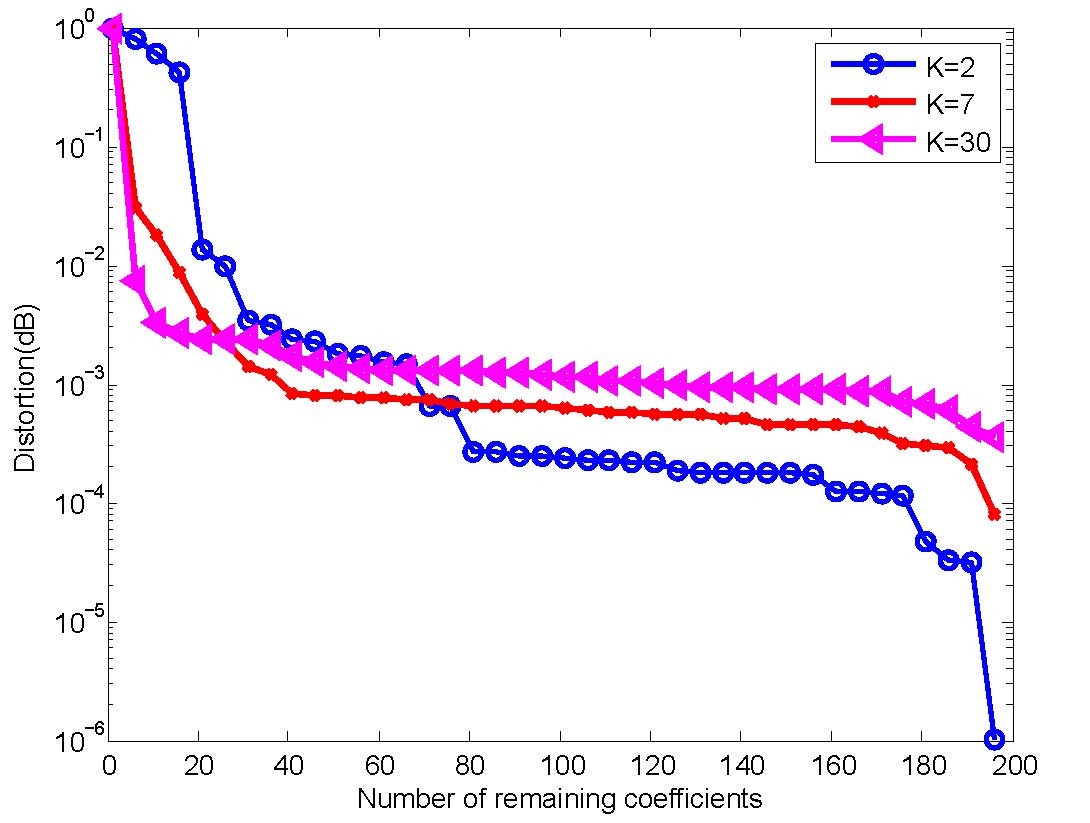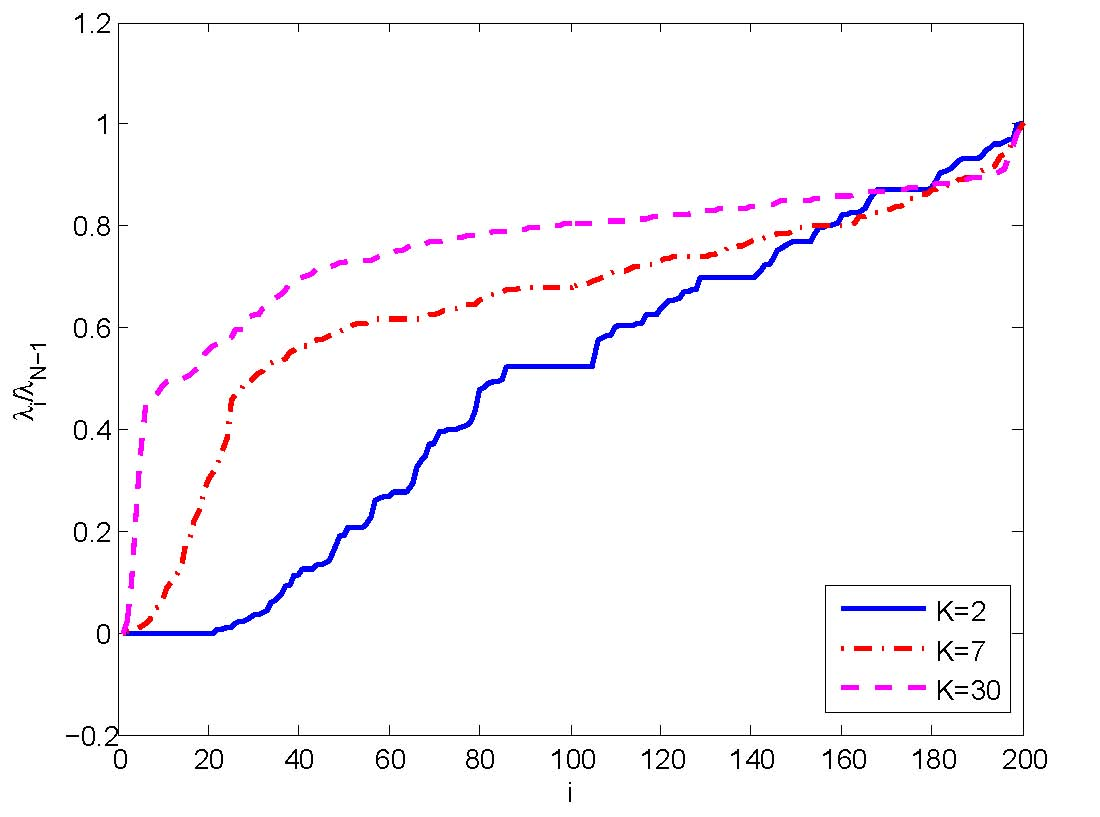Numerical experiments verify our theoretical study. We connect the nodes with similar value via a KNN graph to make the signal smooth on that graph. We change the parameter K to see how the distribution of eigenvalues affects the linear approximation error. The above left figure shows the linear approximation error while the right one illustrates the distribution of normalized eigenvalues.

Graph Spectral Compressed Sensing:

Via previous study, we know that we can obtain compressible signal with regard to the proper GFT basis. Based on such knowledge, we developed a new technique called Graph Spectral Compressed Sensing (GSCS). We show that for a smooth signal with respect to the graph topology, we can gather measurements from a random subset of nodes and then obtain a stable recovery with respect to the graph Laplacian eigenbasis, leveraging ideas from compressed sensing. Moreover, we further show that GSCS can be applied to spatially or temporally correlated signals in wireless sensor network.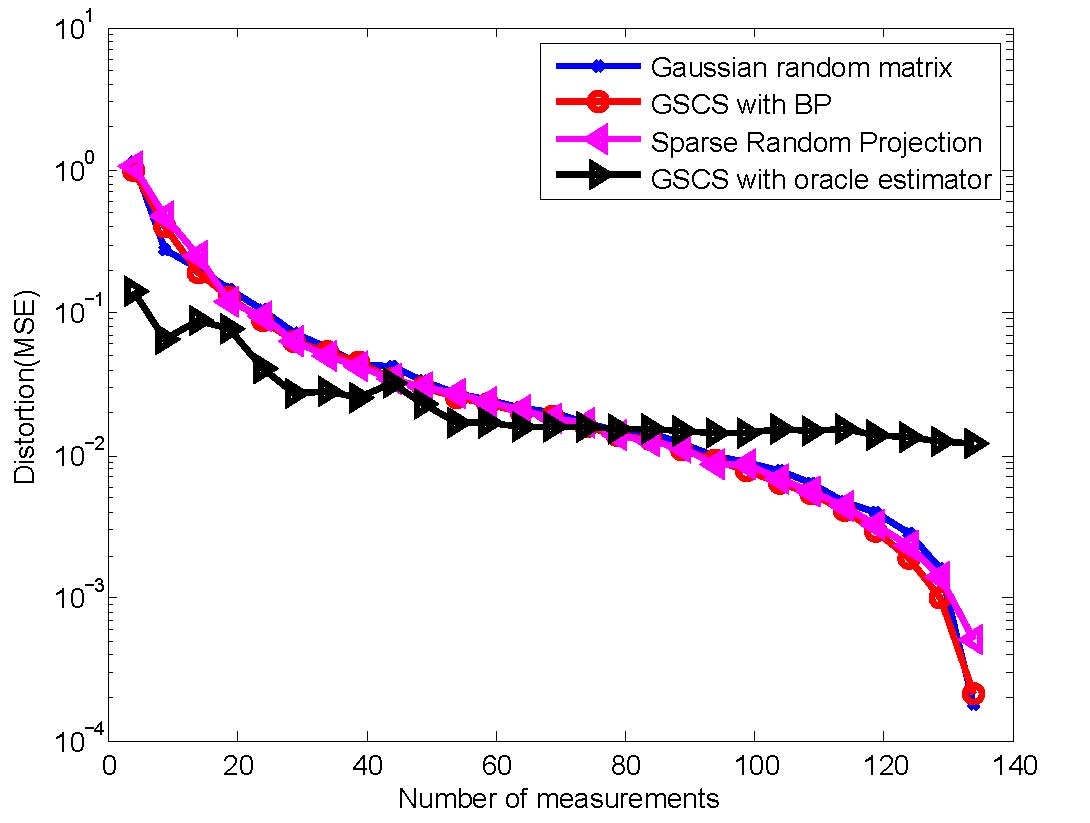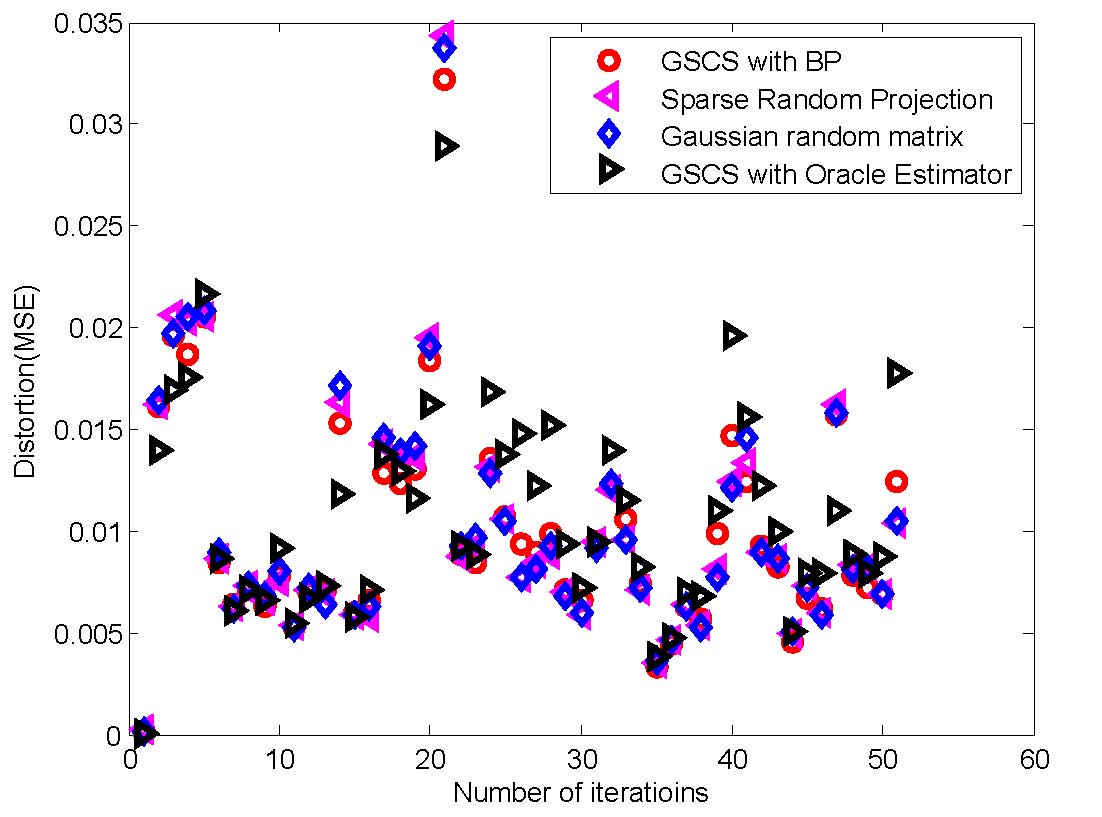The above left figure shows the performance of GSCS on spatially correlated signals and we can see that with less than 40% information, we can still achieve a small distortion and GSCS with oracle estimator performs better than other methods when the number of measurements are small. The above right figure illustrates the online estimation error of GSCS on temporally correlated signals. We merely sample around 35% nodes while still can achieve a very small average MSE around 1%.

People:

•  Faculty: Michael Rabbat
• Master Student: Xiaofan Zhu

Related Publication:

1. X. Zhu and M. Rabbat, Approximating signals supported on graphs, to appear, 2012 IEEE International Conference on Acoustics, Speech, and Signal Processing (ICASSP), March, 2012.
2. X. Zhu and M. Rabbat, Graph spectral compressed sensing for sensor networks, to appear, 2012 IEEE International Conference on Acoustics, Speech, and Signal Processing (ICASSP), March, 2012.